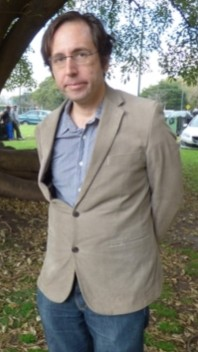# New Take on an Ancient Method Improves Way to Find Prime Numbers

Matias Loewy in Scientific American:Peruvian mathematician Harald Helfgott gained worldwide attention in 2013 when he solved a 271-year-old problem: the so-called Goldbach’s weak conjecture, according to which every odd number greater than 5 can be expressed as the sum of three prime numbers—such as: 2 + 3 + 5 = 11 and 19 + 13 + 3 = 35.

But Helfgott, 38, went even farther back in time and conceived an improved version of the sieve of Eratosthenes, a popular method for finding prime numbers that was formulated circa 240 B.C. Helfgott’s proposed version would reduce the requirement of physical space in computer memory, which in turn would reduce the execution time of programs designed to make that calculation.

Prime numbers are something like “atoms of mathematics,” which can only be divided by themselves and the number 1. Eratosthenes of Cyrene—a Greek mathematician, astronomer and geographer who was director of the Library of Alexandria and became famous for calculating the circumference of Earth—also proposed a practical method to identify them: the “sieve,” or filter. “Like many other children, I was taught this it in primary school when I was 10, with a table,” says Helfgott, who is currently a researcher at the National Center for Scientific Research (CNRS) and the University of Göttingen.

In order to determine with this sieve all primes between 1 and 100, for example, one has to write down the list of numbers in numerical order and start crossing them out in a certain order: first, the multiples of 2 (except the 2); then, the multiples of 3, except the 3; and so on, starting by the next number that had not been crossed out. The numbers that survive this procedure will be the primes. The method can be formulated as an algorithm and computers can quickly run it.

More here.